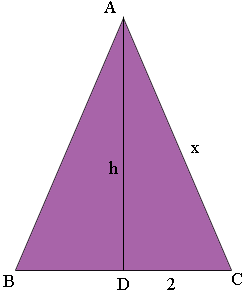SEARCH HOMEMath Central Quandaries & QueriesQuestion from Rita, a student: Express the area A of an isosceles right triangle as a function of x of one of the two equal sides. NOTE: I always see the phrase "Express as a function of x" in math textbooks. What exactly does that phrase mean? I also have seen it written as "Express in terms of x."Hi Rita,

Let me do an example.

An isosceles triangle has a base of length 4 inches and the other two sides have equal length x inches. Write the area a a function of x.

What this is asking is for you to write a function A(x) which gives the area of the triangle and the variable in the function is x.

I drew a diagram and labeled the triangle ABC with |BC| = 4 inches.I let D be the midpoint of BC and then |AD| = h, the height of the triangle and hence the area of the triangle is

1/2 × |BC| × h = 2 × h

Triangle ADC is a right triangle and hence by Pythagoras theorem

h2 + 22 = x2 or h = √(x2 - 4)

Thus the area of the triangle is

2 × h = 2 × √(x2 - 4)

Hence I have what was requested

A(x) = 2 √(x2 - 4)

the area written as a function of x or, the area expressed in terms of x.

I hope this helps,
PennyMath Central is supported by the University of Regina and The Pacific Institute for the Mathematical Sciences.